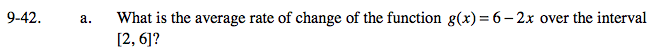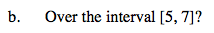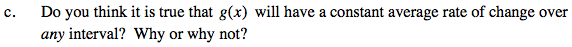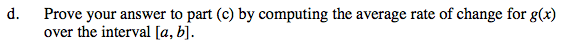### Home > PCT > Chapter Ch9 > Lesson 9.1.3 > Problem9-42

9-42.
1. Complete the following. Homework Help ✎

1. What is the average rate of change of the function g(x) = 6 − 2x over the interval [2, 6]?

2. Over the interval [5, 7]?

3. Do you think it is true that g(x) will have a constant average rate of change over any interval? Why or why not?

4. Prove your answer to part (c) by computing the average rate of change for g(x) over the interval [a, b].$\frac{g(6) - g(2)}{6-2}$$\frac{g(7) - g(5)}{7-5}$Yes, because it is linear.$\frac{g(b) - g(a)}{b-a} = \frac{6 - 2b - (6 - 2a)}{b - a} =$